# Excel VLOOKUP VS HLOOKUPIn this blog we will learn about the difference between VLOOKUP VS HLOOKUP

What is VLOOKUP?

The V in VLOOKUP stands for vertical search (in a single column),

Excel searches for a lookup value in the leftmost column of a section of your spreadsheet called the table array.

The function returns another value in the same row, defined by the column index number.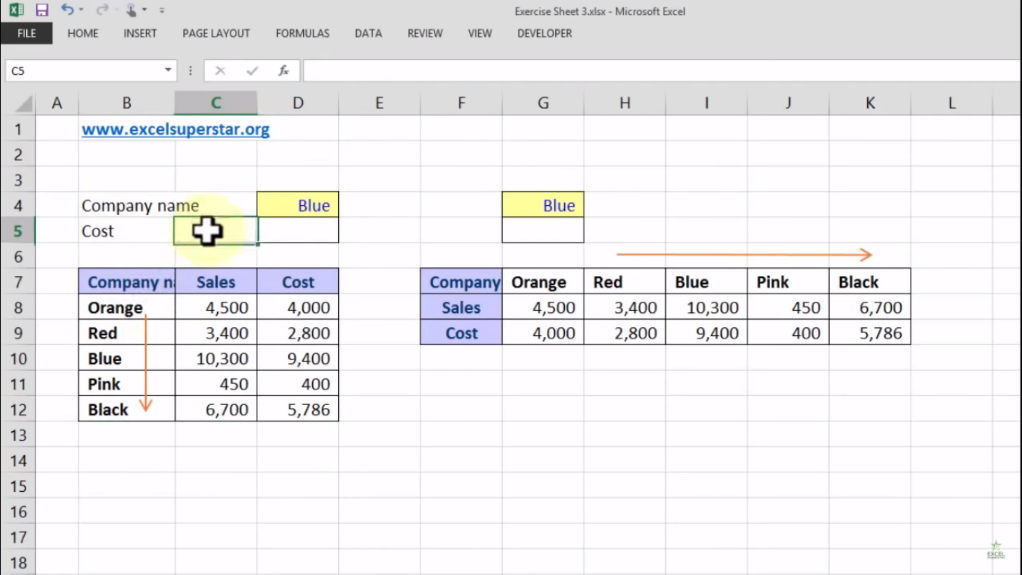The Table explains the following things:

1. From Top to Bottom, the Company Name column is represented by the name of colors like Orange, Red, Pink, Blue and Black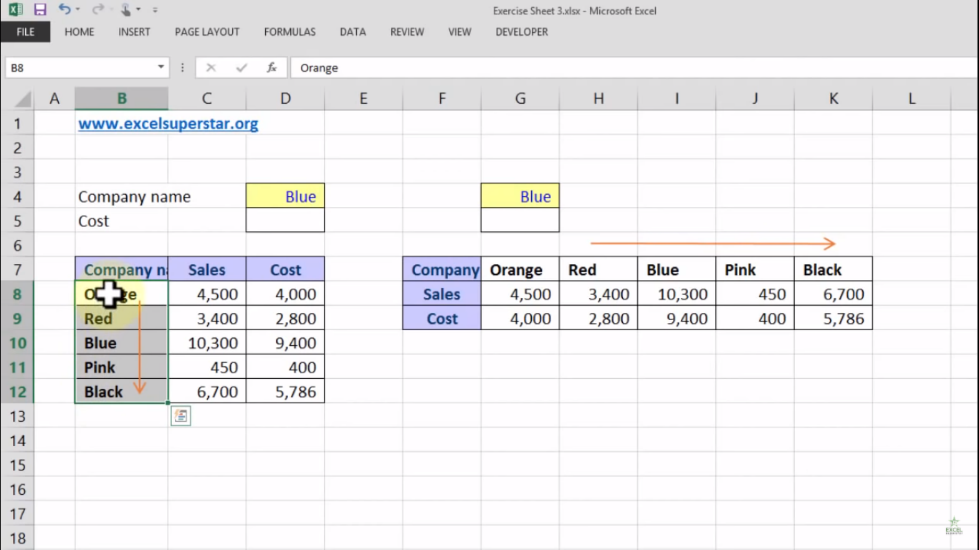2. The Sales column represents the number of sales that are done by each company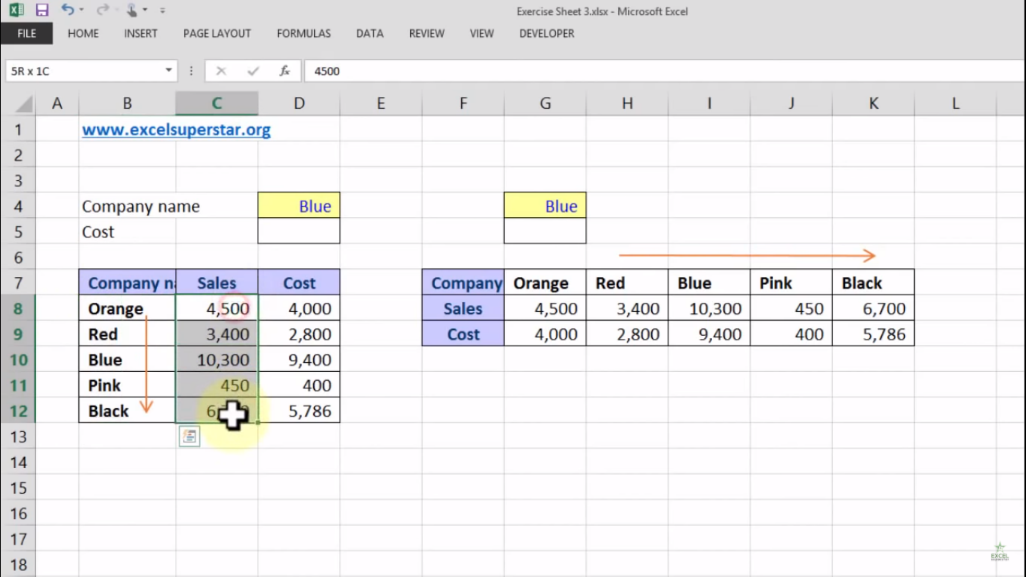3. The Cost column represents the total expenses done by the company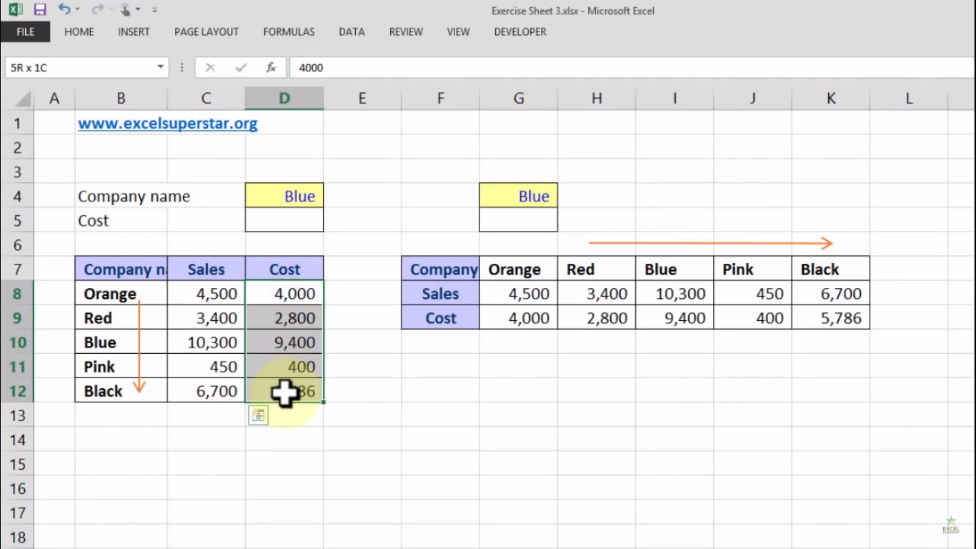Now I want to calculate the cost of Blue color Company from the given table. So here we will apply first VLOOKUP formula and then HLOOKUP Formula to calculate the cost

1. Write =VLOOKUP and press the Tab Key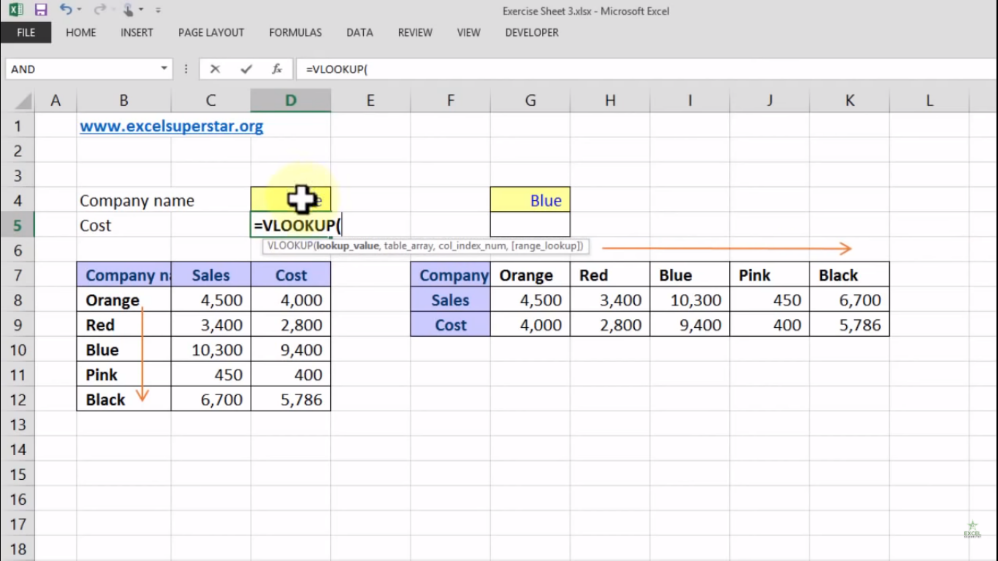The VLOOKUP syntax has the following arguments:

1. value – The value to look for in the first column of a table.

2. table – The table from which to retrieve a value.

3. col_index – The column in the table from which to retrieve a value.

4. range_lookup – [optional] TRUE = approximate match (default). FALSE = exact match.

2. Click on the Blue Color cell =VLOOKUP[D4,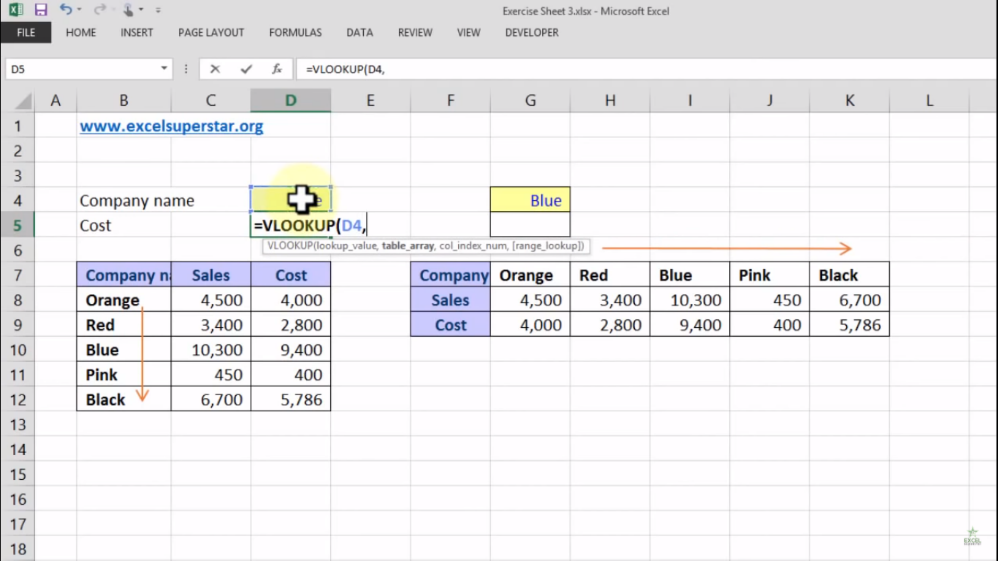3. Choose the entire table and press F4 =VLOOKUP[D4,\$B\$7:\$D\$12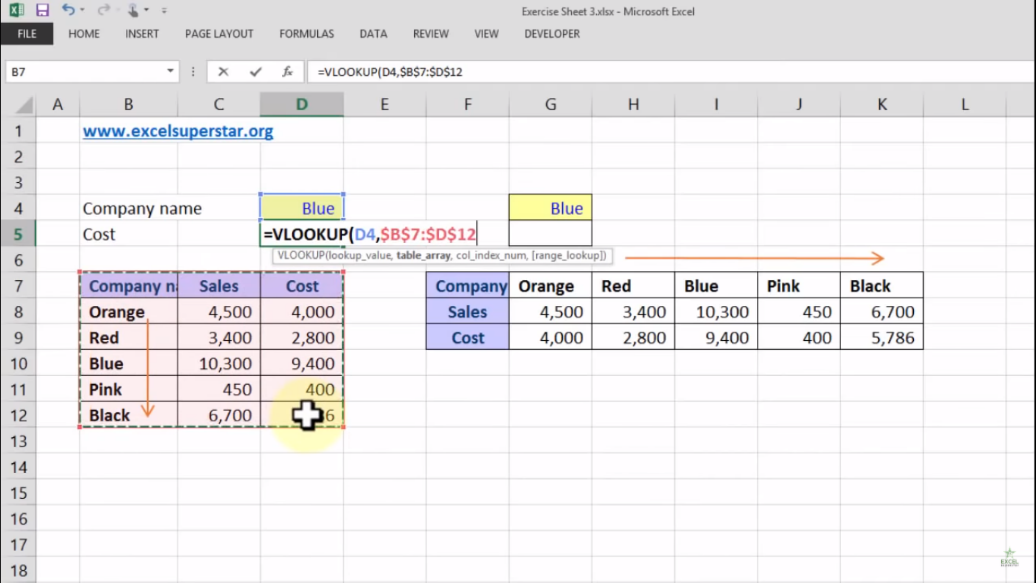4. Write 3 as we want to retrieve a value from 3rd column in the table =VLOOKUP[D4,\$B\$7:\$D\$12,3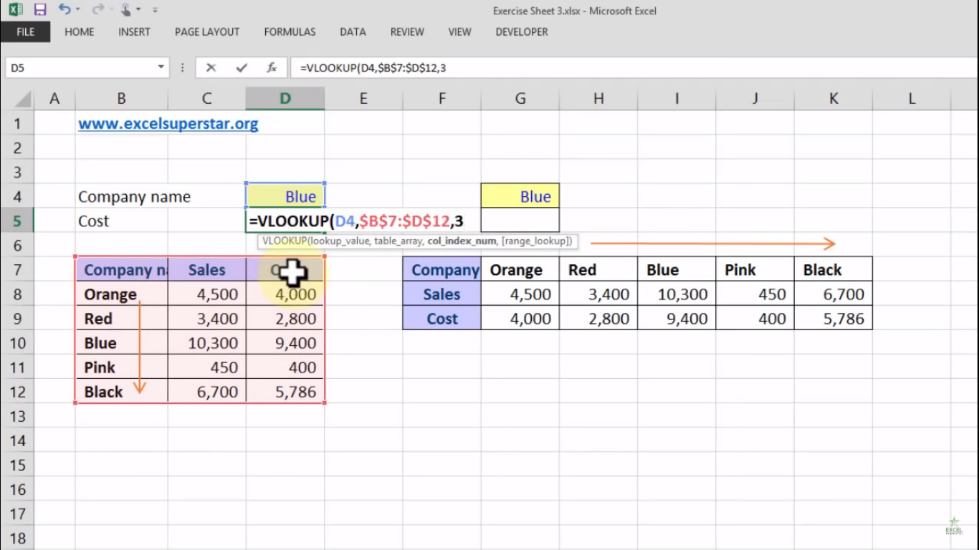5. Write FALSE as an exact match = VLOOKUP[D4,\$B\$7:\$D\$12,3,FALSE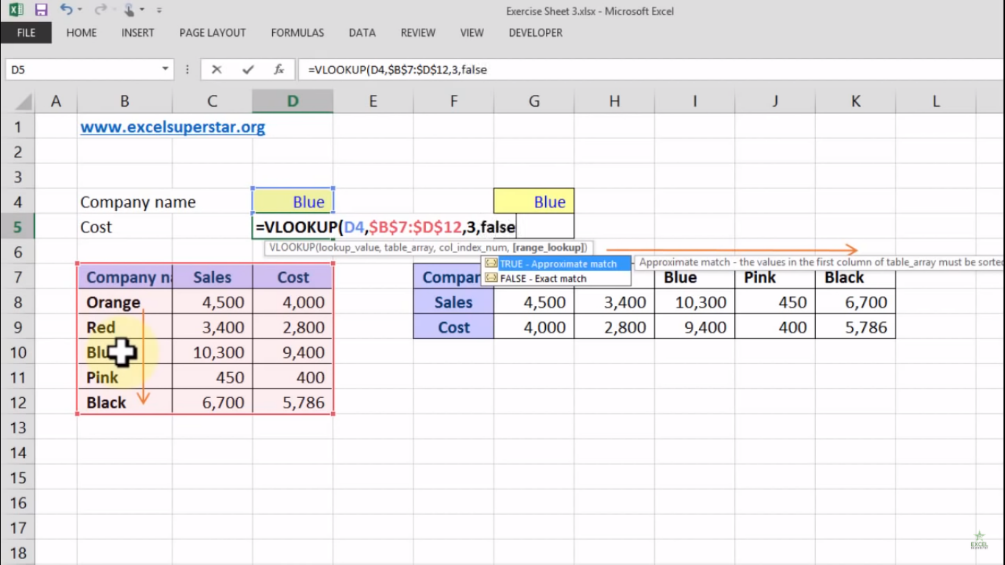Note – If you want then you can write 0 instead of false in the VLOOKUP formula to find the answer

6. Press Enter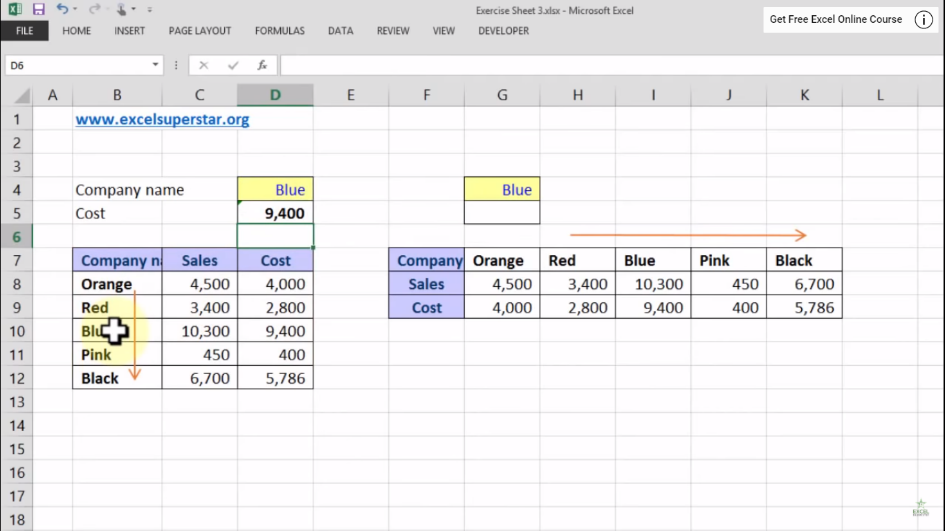Now if you want to transpose the data, then follow the steps given below:

1. Select a cell and right-click on it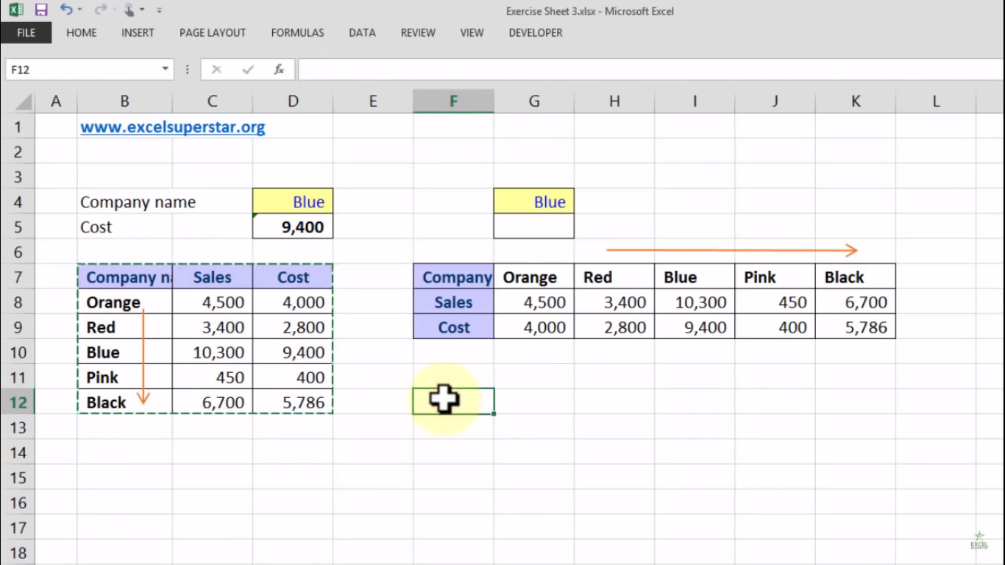2. Right-click on it select Paste Special option3. A Paste Special Panel will open, click on Transpose option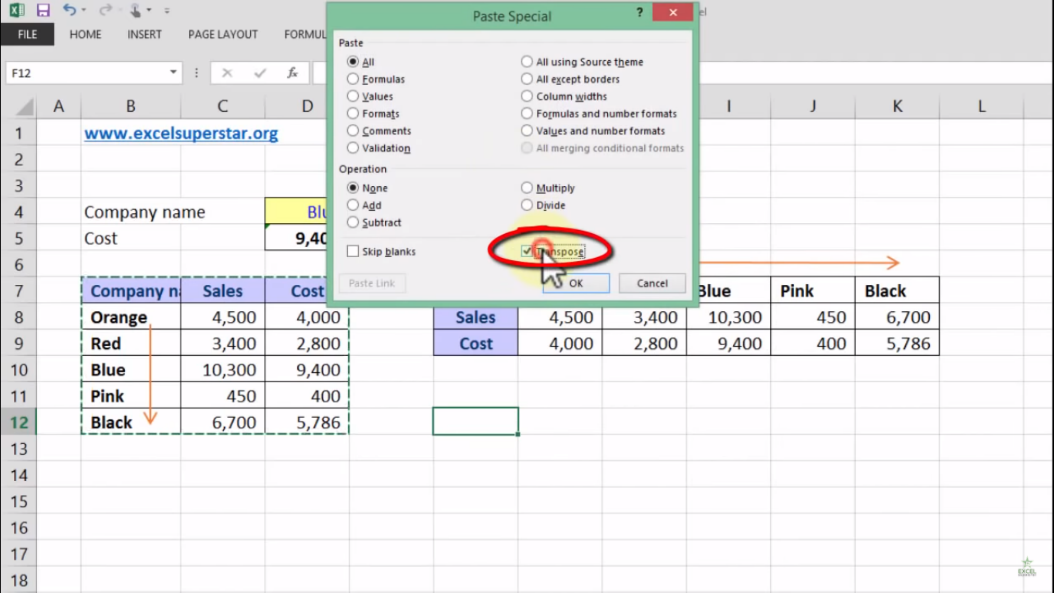4. Click OK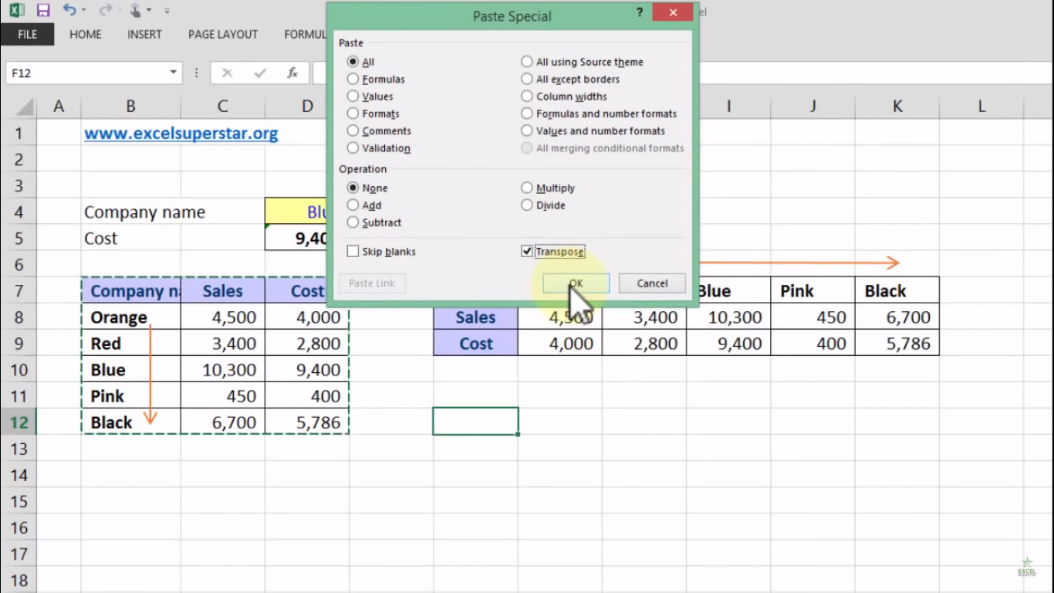What is HLOOKUP?

H in HLOOKUP stands for horizontal search (within a single row).

HLOOKUP is similar to VLOOKUP, but searches a row instead of a column, and the result is offset by a row index number.

1. Write =HLOOKUP and press the Tab Key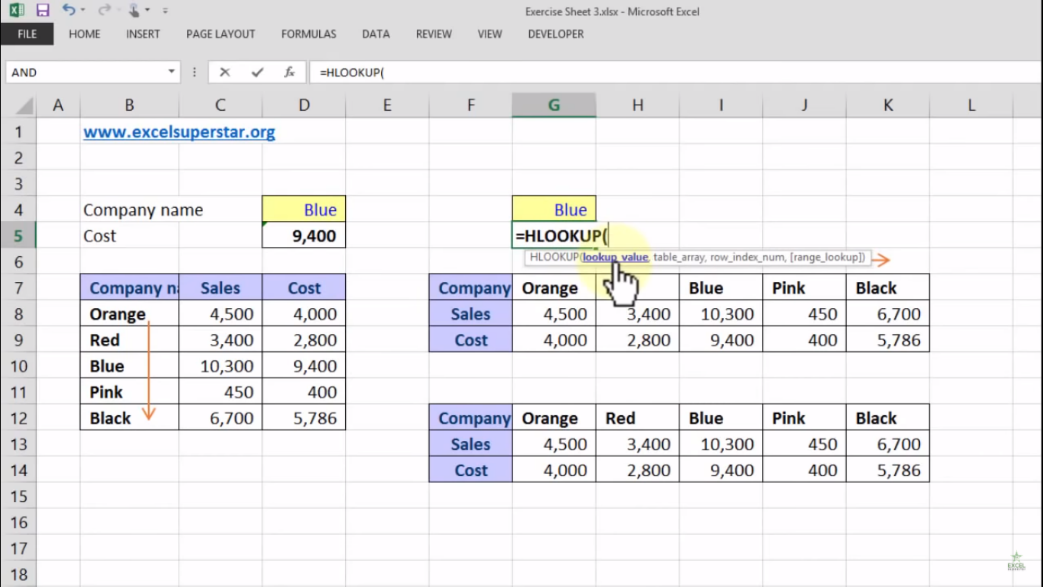2. Select the Blue color cell =HLOOKUP[G4,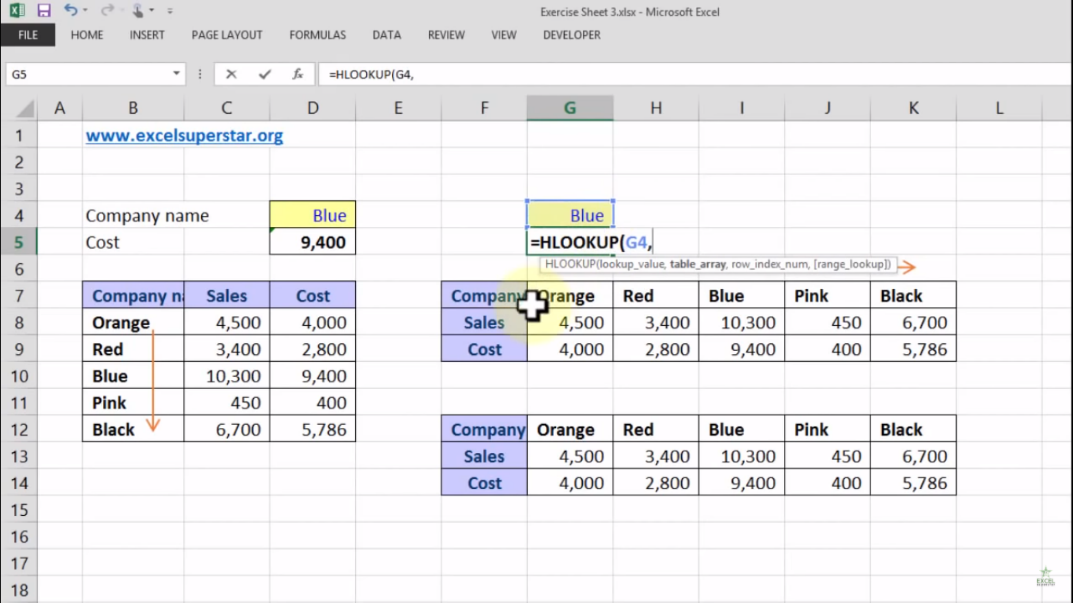3. Choose the entire table and press F4 =HLOOKUP[G4,\$F\$7:\$K\$9,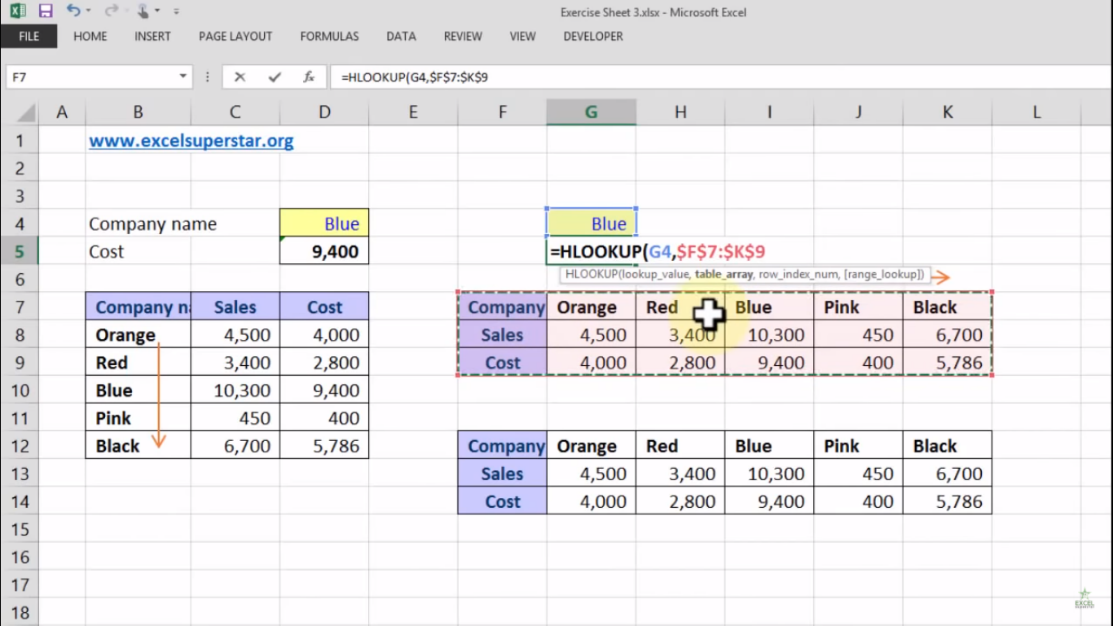5. Write 3 as we want to retrieve a value from 3rd column in the table =HLOOKUP[G4,\$F\$7:\$K\$9,3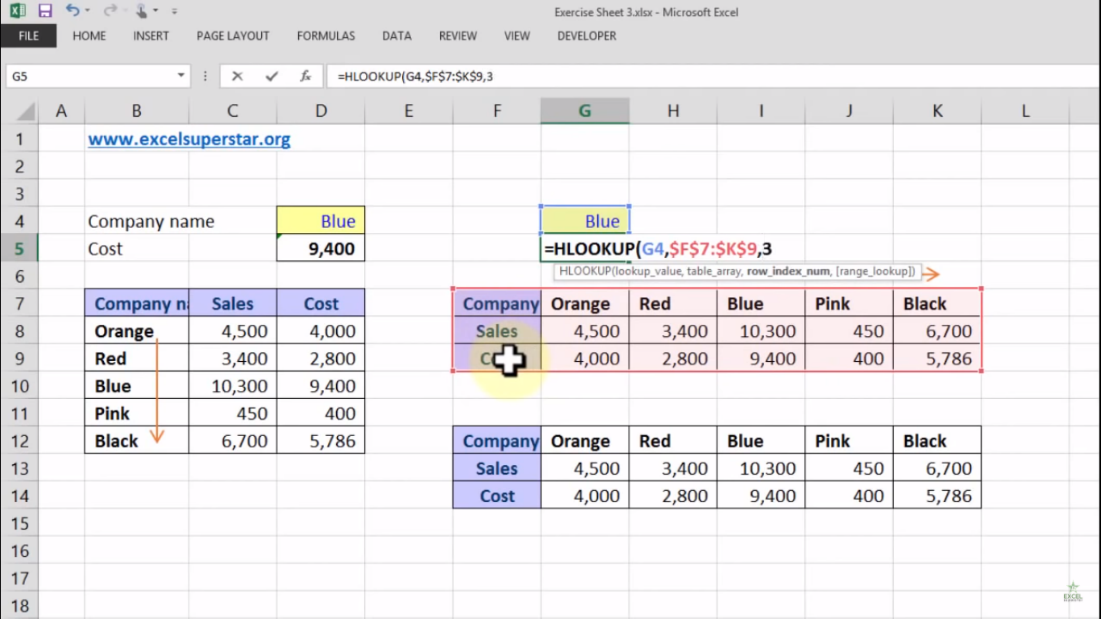6. Write FALSE or 0 as an exact match =HLOOKUP[G4,\$F\$7:\$K\$9,3]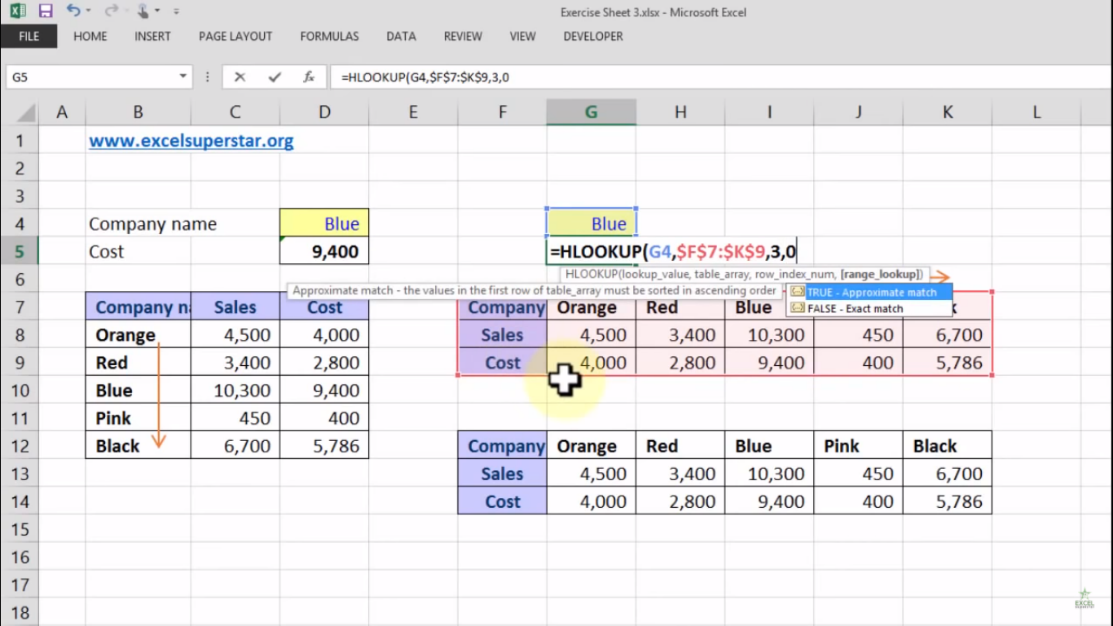7. Press Enter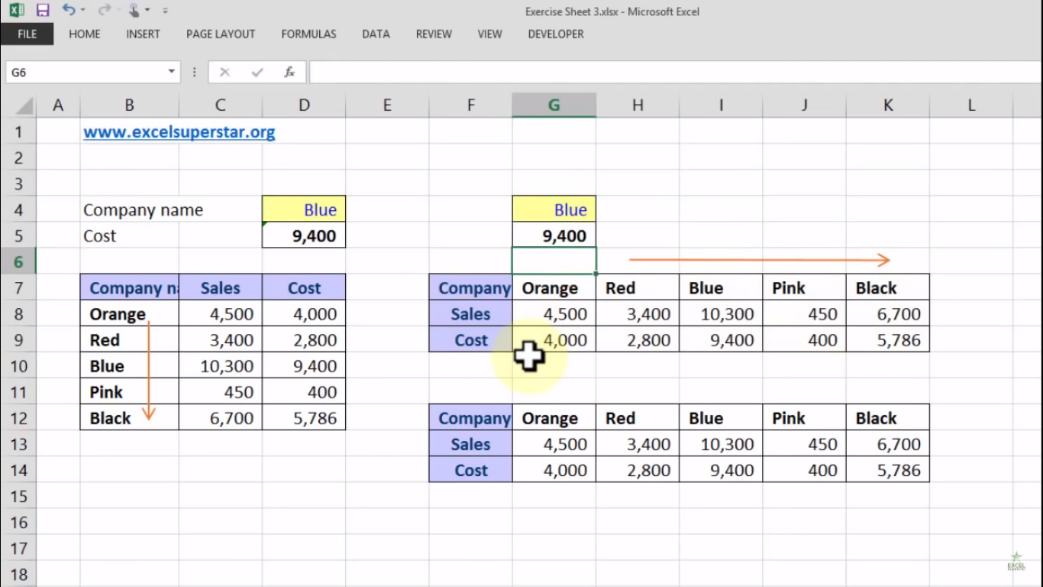Conclusion

Lastly, the difference between VLOOKUP and HLOOKUP Formula is that if the data is placed vertically then it is called as VLOOKUP and if the same ID Codes or Common Links are placed horizontally then it is called as HLOOKUP.